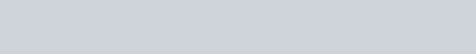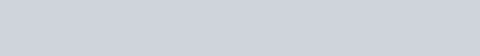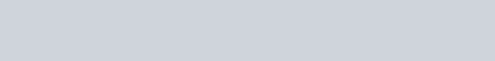# Basics of Strength of materials- (with PDF and PPT)

Hey, are you looking to know about the Basics of strength of materials?

Then you landed on the right platform, here you will learn all about the strength of materials basics.

So, I invite you to come and join the knowledgeable journey with Engineers Rail. The journey which you will never regret in the end.

Before I start, here is a gift for you…

Excited?

Now, you can download this whole article strength of materials basics pdf and strength of materials basics ppt at the end of this session. So, never forget to collect your freebies at the end.

So, without further delays, Let’s get started…

First, let’s start with the basics, and further we will drill down the various concepts of strength of materials, later on down the road.

Classification of Engineering mechanics-

Engineering mechanics is classified into three categories-

• Static
• Dynamic
• Mechanics of materials or strength of materials

### Static and Dynamic mechanics-

Static and dynamic mechanics deals with the external effect on the rigid body with applied load. It can not tell about the internal effect that happens inside the body with applied load. The study of the non-deformative body comes under these mechanics, In general.

### Mechanics of Materials-

Mechanics of materials deals with the Internal effect and deformations that are caused by the applied load. Mechanics of materials are also known as Strength of Materials (SOM).

The study of a deformative body or change in shape with applied load, known as strength of materials and deals with the behavior of stresses and strains on the solid body with an applied load such as beams, columns and shaft.

```Why we study strength of materials-
```

As the name suggests, The study of materials’ strength deals with the strength of materials. It plays a very crucial role in designing the body or components such as engines, machines, bridges, flyovers, cranes, lifts, etc.

This can be used to-

• To evaluate the load applied to the body
• Select the material which can sustain the load

SOM is used to select the material and evaluate the strength of the materials as per the load about to be put on, to prevent the failure of the component with applied over time. As you know, when induced stress is gone beyond the material strength, the failure of the material occurs.

In general, the ability to withstand an applied load without failure or plastic deformation is known as the strength of the material.

Now, Let’s discuss the basics of SOM with its equations.

## Strength of materials basics with equations used-

Here are some basic definitions of SOM along with its equations-

### Stress-

Stress is the internal resisting force developed in the body due to externally applied load. It is further denoted by σ.

The stress formula is given by-Where P= Force.  A= Area and the unit is- N/mm2

### Strain-

When an applied load causes deformation in the body, the deformation per unit length is called strain. It is denoted by ∈.

The strain formula is given by-Where, δl= change in length, l= original length, and there is no unit of the strain.

Stress-strain curve diagram-When the steel specimen is subjected to tensile load, the stress is directly proportional to the strain till the elastic limit, This is also known as Hook’s law.

The constant proportionally is known as young’s modulus law. It presents the slowness of this curve before the elastic limit. Upon loading it further, permanent deformation produces, and this behavior is known as strain hardening.

After further loading, the cross-sectional area of the specimen rapidly decreases and this phenomenon is known as necking.

And finally, the specimen reaches its point and gets fractured into cup and cone shapes.

### Modulus of elasticity-

It is the ratio of stress in the body to the strain applied to the body. The unit of modulus of elasticity is – N/mm2

The formula of Modulus of elasticity given by-### Poisson Ratio-

Poisson’s ratio is the ratio between lateral strain to the linear strain within elastic limit under direct loading. It is denoted by µ or 1/m.

The famous French mathematician Simeon Denis Poisson is the founder of the Poisson’s ratio in 1827.

The formula of Poisson’s ratio is given by-### The volumetric strain of a rectangular body subjected to an axial load-Where, b= width, t=thickness, E=modulus of elasticity, 1/m= Poisson’s ratio

The volumetric strain of a rectangular body subjected to three mutually perpendicular forces-Where, ∈= Strain in x-direction, ∈y= Strain in y-direction, ∈z= strain in z-direction

### Bending Equation-

Destruction in components due to applied load in any of the longitudinal planes is known as bending.

The bending equation is given by-Where, M= bending moment, I= moment of inertia, σ= Bending stress, y= (D/2) distance from the neutral axis, E= modulus of elasticity, R= radius of curvature

### Torsional Equation-θτ

Where, T= torque, J= Polar moment of inertia, C= Torsional Rigidity, θ= Angle of twist, τ= shear stress, R= Radius of the circular shaft.

Here is the video guide to help you go deep into Introduction to the strength of materials

What did you learn?

Today, I have discussed the basics of strength of materials including formulas and their definitions. I hope you enjoyed spending time here and gained something valuable.

If so, share with your near and dear ones including colleagues and friends, students, and aspirants to help them grow as well.

If you have any questions, feel free to ask in the comment section down below or if you have any suggestions to make this place better for you please don’t hesitate to share that valuable feedback with me through various contact mediums available on this website.

And don’t forget to bookmark Engineers Rail for further convenient readings.

Want to read more? Here are some suggested articles, you should read further-

Strength of Materials MCQ

Thank you for being with me. I hope to see you in the next session.

Before you go, Here are your freebies all yours-

 strength of materials basics pptstrength of materials basics pdfshow-on-desktop show-on-mobile